upm  1.7.1 Sensor/Actuator repository for libmraa (v2.0.0)
LSM6DSL Class Reference

API for the LSM6DSL 3-axis Accelerometer and Gyroscope. More...

## Detailed Description

The LSM6DSL is a system-in-package featuring a 3D digital accelerometer and a 3D digital gyroscope performing at 0.65 mA in high performance mode and enabling always-on low-power features for an optimal motion experience for the consumer.

Not all functionality of this chip has been implemented in this driver, however all the pieces are present to add any desired functionality. This driver supports both I2C (default) and SPI operation.

// Instantiate an LSM6DSL using default I2C parameters
upm::LSM6DSL sensor;
// For SPI, bus 0, you would pass -1 as the address, and a valid pin
// for CS: LSM6DSL(0, -1, 10);
// now output data every 250 milliseconds
while (shouldRun) {
float x, y, z;
sensor.update();
sensor.getAccelerometer(&x, &y, &z);
cout << "Accelerometer x: " << x << " y: " << y << " z: " << z << " g" << endl;
sensor.getGyroscope(&x, &y, &z);
cout << "Gyroscope x: " << x << " y: " << y << " z: " << z << " dps" << endl;
// we show both C and F for temperature
cout << "Compensation Temperature: " << sensor.getTemperature() << " C / "
<< sensor.getTemperature(true) << " F" << endl;
cout << endl;
upm_delay_us(250000);
}

## Public Member Functions

LSM6DSL (int bus=LSM6DSL_DEFAULT_I2C_BUS, int addr=LSM6DSL_DEFAULT_I2C_ADDR, int cs=-1)

virtual ~LSM6DSL ()

void update ()

uint8_t getChipID ()

void getAccelerometer (float *x, float *y, float *z)

std::vector< float > getAccelerometer ()

void getGyroscope (float *x, float *y, float *z)

std::vector< float > getGyroscope ()

float getTemperature (bool fahrenheit=false)

void init (LSM6DSL_XL_ODR_T acc_odr=LSM6DSL_XL_ODR_104HZ, LSM6DSL_XL_FS_T acc_fs=LSM6DSL_XL_FS_2G, LSM6DSL_G_ODR_T gyr_odr=LSM6DSL_G_ODR_104HZ, LSM6DSL_G_FS_T gyr_fs=LSM6DSL_G_FS_245DPS)

void reset ()

void setAccelerometerODR (LSM6DSL_XL_ODR_T odr)

void setAccelerometerFullScale (LSM6DSL_XL_FS_T fs)

void setGyroscopeODR (LSM6DSL_G_ODR_T odr)

void setGyroscopeFullScale (LSM6DSL_G_FS_T fs)

void setHighPerformance (bool enable)

void setInterruptActiveHigh (bool high)

void setInterruptPushPull (bool pp)

uint8_t getStatus ()

void setAccelerometerOffsets (int x, int y, int z, bool weight)

void installISR (LSM6DSL_INTERRUPT_PINS_T intr, int gpio, mraa::Edge level, void(*isr)(void *), void *arg)

void uninstallISR (LSM6DSL_INTERRUPT_PINS_T intr)

uint8_t readReg (uint8_t reg)

int readRegs (uint8_t reg, uint8_t *buffer, int len)

void writeReg (uint8_t reg, uint8_t val)

## Protected Attributes

lsm6dsl_context m_lsm6dsl

## Constructor & Destructor Documentation

 LSM6DSL ( int bus = `LSM6DSL_DEFAULT_I2C_BUS`, int addr = `LSM6DSL_DEFAULT_I2C_ADDR`, int cs = `-1` )

LSM6DSL constructor.

This device can support both I2C and SPI. For SPI, set the addr to -1, and specify a positive integer representing the Chip Select (CS) pin for the cs argument. If you are using a hardware CS pin (like edison with arduino breakout), then you can connect the proper pin to the hardware CS pin on your MCU and supply -1 for cs. The default operating mode is I2C.

Parameters
 bus I2C or SPI bus to use addr The address for this device. -1 for SPI cs The gpio pin to use for the SPI Chip Select. -1 for I2C or for SPI with a hardware controlled pin.
Exceptions
 std::runtime_error on initialization failure
 ~LSM6DSL ( )
virtual

LSM6DSL destructor

Here is the call graph for this function: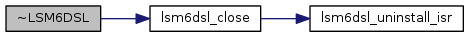## Member Function Documentation

 void update ( void )

Update the internal stored values from sensor data

Exceptions
 std::runtime_error on failure

Here is the call graph for this function: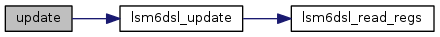uint8_t getChipID ( )

Return the chip ID

Returns
The chip ID

Here is the call graph for this function: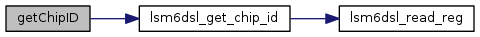void getAccelerometer ( float * x, float * y, float * z )

Return accelerometer data in gravities. update() must have been called prior to calling this method.

Parameters
 x Pointer to a floating point value that will have the current x component placed into it y Pointer to a floating point value that will have the current y component placed into it z Pointer to a floating point value that will have the current z component placed into it

Here is the call graph for this function: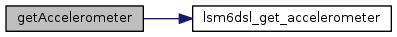std::vector< float > getAccelerometer ( )

Return accelerometer data in gravities in the form of a floating point vector. update() must have been called prior to calling this method.

Returns
A floating point vector containing x, y, and z in that order
 void getGyroscope ( float * x, float * y, float * z )

Return gyroscope data in degrees per second (DPS). update() must have been called prior to calling this method.

Parameters
 x Pointer to a floating point value that will have the current x component placed into it y Pointer to a floating point value that will have the current y component placed into it z Pointer to a floating point value that will have the current z component placed into it

Here is the call graph for this function: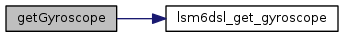std::vector< float > getGyroscope ( )

Return gyroscope data in degrees per second in the form of a floating point vector. update() must have been called prior to calling this method.

Returns
A floating point vector containing x, y, and z in that order
 float getTemperature ( bool fahrenheit = `false` )

Return the current measured temperature. Note, this is not ambient temperature. update() must have been called prior to calling this method.

Parameters
 fahrenheit true to return data in Fahrenheit, false for Celicus. Celsius is the default.
Returns
The temperature in degrees Celsius or Fahrenheit

Here is the call graph for this function: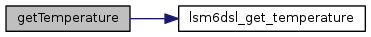void init ( LSM6DSL_XL_ODR_T acc_odr = `LSM6DSL_XL_ODR_104HZ`, LSM6DSL_XL_FS_T acc_fs = `LSM6DSL_XL_FS_2G`, LSM6DSL_G_ODR_T gyr_odr = `LSM6DSL_G_ODR_104HZ`, LSM6DSL_G_FS_T gyr_fs = `LSM6DSL_G_FS_245DPS` )

Initialize the device and start operation. This function is called from the constructor, so it will not need to be called by a user unless the device is reset. It sets the accelerometer and gyroscope ODR and FS modes, and enables BDU, register auto-increment, and high performance mode.

Parameters
 acc_odr One of the LSM6DSL_XL_ODR_T values acc_fs One of the LSM6DSL_XL_FS_T values gyr_odr One of the LSM6DSL_G_ODR_T values gyr_fs One of the LSM6DSL_G_FS_T values
Exceptions
 std::runtime_error on failure

Here is the call graph for this function: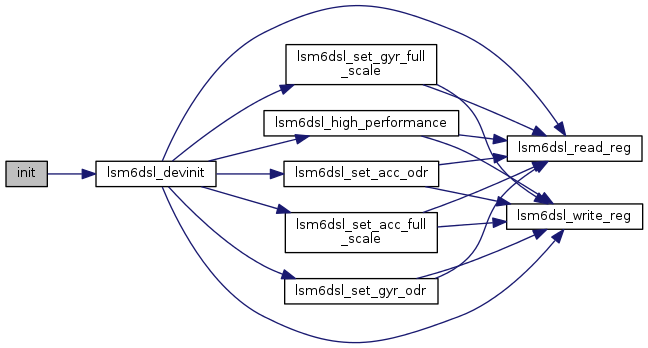void reset ( )

Reset the device as if during a power on reset. All configured values are lost when this happens. You should call init() afterwards, or at least perform the same initialization init() does before continuing.

Exceptions
 std::runtime_error on failure

Here is the call graph for this function:void setAccelerometerODR ( LSM6DSL_XL_ODR_T odr )

Set the output data rate (ODR) of the accelerometer

Parameters
 odr One of the LSM6DSL_XL_ODR_T values
Exceptions
 std::runtime_error on failure

Here is the call graph for this function:void setAccelerometerFullScale ( LSM6DSL_XL_FS_T fs )

Set the full scale (FS) of the accelerometer. This device supports a full scale of 2, 4, 8, and 16G.

Parameters
 fs One of the LSM6DSL_XL_FS_T values
Exceptions
 std::runtime_error on failure

Here is the call graph for this function:void setGyroscopeODR ( LSM6DSL_G_ODR_T odr )

Set the output data rate (ODR) of the gyroscope

Parameters
 odr One of the LSM6DSL_G_ODR_T values
Exceptions
 std::runtime_error on failure

Here is the call graph for this function:void setGyroscopeFullScale ( LSM6DSL_G_FS_T fs )

Set the full scale (FS) of the gyroscope

Parameters
 fs One of the LSM6DSL_G_FS_T values
Exceptions
 std::runtime_error on failure

Here is the call graph for this function:void setHighPerformance ( bool enable )

Enable accelerometer and gyroscope high performance modes. These are further defined by the respective ODR settings to allow low power, and normal/high-performance modes. This is enabled by default in init().

Parameters
 enable true to enable high performance mode, false otherwise
Exceptions
 std::runtime_error on failure

Here is the call graph for this function:void setInterruptActiveHigh ( bool high )

Indicate whether the interrupt should be active high (default) or active low. See the datasheet for details.

Parameters
 high true for active high, false for active low
Exceptions
 std::runtime_error on failure

Here is the call graph for this function:void setInterruptPushPull ( bool pp )

Indicate whether interrupts are push-pull (default) or open drain. See the datasheet for details.

Parameters
 pp true for push-pull, false for open-drain
Exceptions
 std::runtime_error on failure

Here is the call graph for this function:uint8_t getStatus ( )

Return the contents of the status register

Returns
A bitmask of values from LSM6DSL_STATUS_BITS_T

Here is the call graph for this function: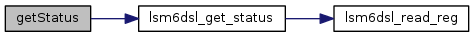void setAccelerometerOffsets ( int x, int y, int z, bool weight )

Set accelerometer offsets for each axis. In the case of X and Y, the offsets will be internally added before being placed into the output registers. For the Z offset, the value will be subtracted before being placed into the output registers. The weight is used to determine the weighing of the offset bits. All offsets must be in the range of -127 to 127.

Parameters
 x X axis offset in the range -127 to 127 y Y axis offset in the range -127 to 127 z Z axis offset in the range -127 to 127 weight When true, the the offset will be weighted at 2^-6 g/LSB, when false the weighting will be 2^-10 g/LSB
Exceptions
 std::runtime_error on failure

Here is the call graph for this function:void installISR ( LSM6DSL_INTERRUPT_PINS_T intr, int gpio, mraa::Edge level, void(*)(void *) isr, void * arg )

install an interrupt handler

Parameters
 intr One of the LSM6DSL_INTERRUPT_PINS_T values specifying which interrupt pin you are installing gpio GPIO pin to use as interrupt pin level The interrupt trigger level (one of mraa::Edge values). Make sure that you have configured the interrupt pin properly for whatever level you choose. isr The interrupt handler, accepting a void * argument arg The argument to pass the the interrupt handler
Exceptions
 std::runtime_error on failure

Here is the call graph for this function: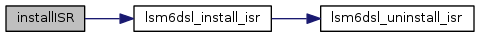void uninstallISR ( LSM6DSL_INTERRUPT_PINS_T intr )

uninstall a previously installed interrupt handler

Parameters
 intr One of the LSM6DSL_INTERRUPT_PINS_T values specifying which interrupt pin you are removing

Here is the call graph for this function: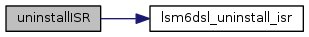uint8_t readReg ( uint8_t reg )

Parameters
 reg The register to read
Returns
The value of the register

Here is the call graph for this function: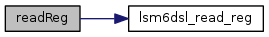int readRegs ( uint8_t reg, uint8_t * buffer, int len )

Read contiguous registers into a buffer

Parameters
 buffer The buffer to store the results len The number of registers to read
Returns
The number of bytes read
Exceptions
 std::runtime_error on failure

Here is the call graph for this function: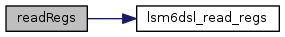void writeReg ( uint8_t reg, uint8_t val )

Write to a register

Parameters
 reg The register to write to val The value to write
Exceptions
 std::runtime_error on failure

Here is the call graph for this function: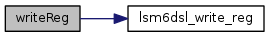Collaboration diagram for LSM6DSL: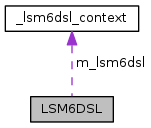[legend]

The documentation for this class was generated from the following files: